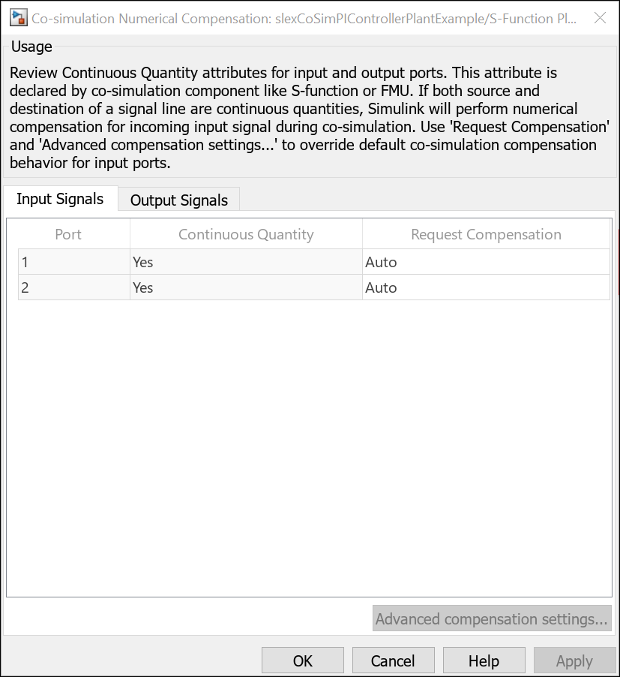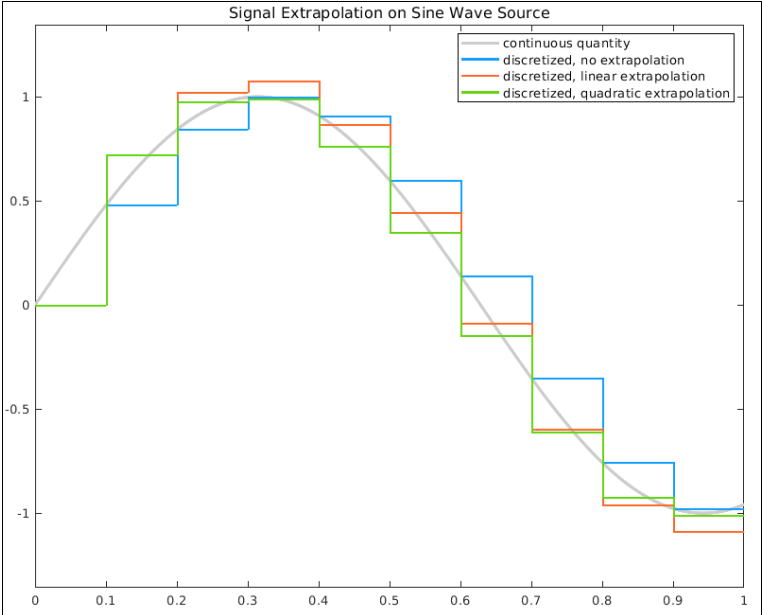## Numerical Compensation

Cosimulation signals typically represent continuous physical quantities that are discretized due to cosimulation. Data exchanges between cosimulation components such as C MEX S-functions and Co-Simulation FMU blocks can introduce numerical inaccuracies from signal delays. Use numerical compensation to improve numerical behavior for simulation involving components that use their own solver. Model Advisor includes a check that detects cosimulation components and advises numerical compensation.

### Numerical Compensation Prerequisites

Simulink® automatically performs numerical compensation for cosimulation signals between cosimulation components. Simulink performs numerical compensation at the input of the destination block. A signal is automatically qualified for numerical compensation if its source port and destination port satisfy these conditions:

• The source port for the signal must satisfy these requirements:

• S-Function

• Output port data type is double

• Output port sample time is periodic and discrete

• Output port complexity is real

• `ssSetOutputPortIsContinuousQuantity()` is set to `true` for the port

• FMU

• Output port data type is double

• FMU is in cosimulation mode

• Block sample time is periodic and discrete

• Output port maps to a variable with `variability='continuous'` in `modelDescription.xml`

• The destination port for the signal must satisfy these requirements:

• S-Function

• Input port data type is double

• Input port sample time is periodic and discrete

• Input port complexity is real

• `ssSetInputPortIsContinuousQuantity()` is set to `true` for this port

• `ssSetInputPortDirectFeedThrough()` is set to `false` for this port

• FMU

• Input port data type is double

• FMU is in cosimulation mode

• Block sample time is periodic and discrete

• Input port maps to a variable with `variability='continuous'` in `modelDescription.xml`

For an example of identifying cosimulation signals for numerical compensation, see Using Numerical Compensation for Co-Simulation Integration .

### Manage Numerical Compensation Options Using the Dialog

When Simulink detects signals that can have numerical compensation, the corresponding input ports display theicon.

The `CoSimPIControllerPlantExample` example includes cosimulation signals that can have numerical compensation:1. Open the `slexCoSimPIControllerPlantExample` model.

`openExample('slexCoSimPIControllerPlantExample')`
2. Update the diagram. Simulink detects the signals that can have numerical compensation, and marks the corresponding ports with theicon.3. Adjust the parameters for automatic compensation using one of these methods:

• Right-click the icon and select Co-simulation Numerical Compensation.

• Right-click the FMU or S-Function block and select Co-simulation Numerical Compensation.

Note

The FMU block enables this option only if it is a block that can potentially support cosimulation, including these blocks:

• S-Function

• FMU Import

• MATLAB System

• Model

• Subsystem

The cosimulation compensation configuration dialog displays Continuous Quantity and Request Compensation attributes for input signals and Continuous Quantity attribute for output signals.1. On the Input Signals tab, select the port for which you want to adjust the automatic compensation.

2. The Continuous Quantity column verifies if the component can support automatic numerical compensation.

3. Double-click the Request Compensation column and select the automatic compensation.

SettingBehaviorIcon

`Auto`

Enable automatic numerical compensation, allowing Simulink to detect if the port has a signal eligible for numerical compensation.

Continuous Quantity for both source and destination signal must be set to `Yes`.`Off`

Disable automatic numerical compensation. Icon appears with a red slash.

Continuous Quantity is ignored.`Always`

Force the port to be considered compliant for numerical compensation, even if the signal is not eligible for numerical compensation. This setting lets you add compensation without declaring it to be continuous.

Continuous Quantity is ignored.4. Adjust the parameters for compensation accuracy. Right-click the icon and select Co-simulation Numerical Compensation.

1. On the Input Signals tab, select the port for which you want to adjust the calculation parameters, then click Advanced compensation settings:

• Extrapolation Method — This method computes a compensated signal value for the current time step of simulation using extrapolation of simulation signal values generated from previous time steps. Select from the three types of extrapolations.

• `Linear` is the default and uses signal values of the previous two time steps to linearly estimate the signal value for use in the current time step of simulation.

• `Quadratic` uses signal values of the previous three time steps to fit the data to a quadratic polynomial.

• `Cubic` uses signal values of the previous four time steps to fit the data to a cubic polynomial.In the beginning of the simulation, when there is an insufficient number of past signal values, a lower-order extrapolation method is used automatically. Higher-order extrapolation methods use more past signal values to predict the current signal value and can improve accuracy of the prediction. However, high-order extrapolation methods can also be numerically unstable. The best extrapolation method depends on the nature of the signal and its sample rate, as specified in the Communication Step Size parameter.

• Signal correction coefficient — This method further adjusts the extrapolated signal value based on past simulation results and past estimated signal values. A correction coefficient is offered for selection between `0` and `1`, where `0` means no adjustment is made to the extrapolated signal value. The default setting for the correction coefficient is `1`. For a given extrapolated signal at a given time step, the larger the signal correction coefficient is, the more the given extrapolated signal is adjusted.

2. If numerical compensation is not beneficial, disable it by left-clicking theicon. When disabled, the icon appears with a red slash.

### Manage Numerical Compensation Options from the Command Line

If automatic compensation is not possible, you can manually enable numerical compensation using the `CoSimSignalCompensationMode` property.

The `CoSimSignalCompensationMode` property has these values:

IconSettingBehavior`'Auto'`

Enable automatic numerical compensation, allowing Simulink to detect if the port has a signal eligible for numerical compensation.

Continuous Quantity for both source and destination signal must be set to `Yes`.`'Auto_Off'`

Disable automatic numerical compensation. Icon appears with a red slash.

Continuous Quantity is ignored.`'Always'`

Force the port to be considered numerical compensation compliant, even if the signal is not eligible for numerical compensation. This setting lets you add compensation without declaring it to be continuous.

Continuous Quantity is ignored.`'Always_Off'`

Disable forcing the port to be considered numerical compensation compliant.

Continuous Quantity is ignored.

For example, to disable numerical compensation for the first input port from the previous model:

1. Select the block for which you want to select the port for numerical compensation. For example, get all the port handles for the currently selected block S-Function Plant #1, `gcb`.

`p = get_param(gcb, 'PortHandles')`

This function returns all the ports for the currently selected block. For example,

```p = struct with fields: Inport: [79.0001 24.0001] Outport: 82.0001 Enable: [] Trigger: [] State: [] LConn: [] RConn: [] Ifaction: [] Reset: [] Event: []```
2. To disable numerical compensation for the first port:

```set_param(p.Inport(2), 'CoSimSignalCompensationMode', 'Auto_Off') ```

The associated port appears with a red slash.You can also set signal compensation parameters from the command line. The first step, again, is to obtain the port handles:

`p = get_param(block, 'PortHandles')`

Set the compensation parameters using the `CoSimSignalCompensationConfig` parameter, in this format:

`set_param(p.Inport,'CoSimSignalCompensationConfig','{<CompensationParam>:<ParamValue>}' )`

Find compensation parameter names and possible values in this table:

Compensation parameterParameter nameParameter valueDefault
`ExtrapolationMethod`Extrapolation method`'LinearExtrapolation'`, `'QuadraticExtrapolation'`, or `'CubicExtrapolation'``'LinearExtrapolation'`
`CompensationCoefficient`Signal correction coefficientScalar between `0` and `1``1`

For example, set the extrapolation method for the port:

`set_param(p.Inport, 'CoSimSignalCompensationConfig', '{"ExtrapolationMethod":"LinearExtrapolation"}' ))`

Set both the extrapolation method and the compensation coefficient:

`set_param(p.Inport,'CoSimSignalCompensationConfig', '{"ExtrapolationMethod":"QuadraticExtrapolation", "CompensationCoefficient":"0.7"}' ))`

### Numerical Compensation Limitations

• Nondouble nonreal signals, such as `single`, `logical`, complex, and bus signals cannot be compensated.

• Numerical compensation is not typically reliable when a rate transition exists between a source and destination component.

• Numerical compensation does not support code generation.

• Ensure that the compensated input port is a continuous quantity, especially if you override the behavior with the `Always` setting. Ideally, the input contributes to the integration process of the dynamic system, such as being integrated over time in the local solver, rather than being used as a discrete control signal, or being differentiated.

• If the Model block with local solver is turned on, no compensation is required at the input port.